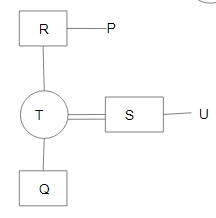Question 21

# $$A + B$$ means ‘B is the brother of A’:$$A - B$$ means ‘A is the mother of B’:$$A \times B$$ means ‘A is the father of B’:$$A \div B$$ means ‘A is the son of B’.If, $$P + R \times T - Q \div S + U$$, then how is S related to R?

Solution

In the diagram, Circle shows the female, square shows the male, vertical lines show the generation, single horizontal line shows the brother/sister and double horizontal lines show the couple.From the diagram,
'S' is son-in-law of 'R'.
$$\therefore$$ The correct answer is option D.